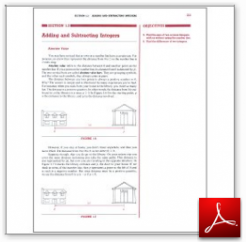Algebra Math Book - Introductory Algebra Thank you for your continual support.

 The power of mathematics rests in the logic of thinking.ID: Sec1-3
Description: Absolute Value-Adding Integers-Difference of Two Integers
Price: .99

# Algebra Math book - Introductory Algebra - Chapter 1 - Section 3 - Adding and Subtracting Integers

## Section 1.3 - Objectives

5.  Find the sum of two or more integers, with or without using the number line.

6.  Find the difference of two integers.

Navigate to
Previous Section or Next Section
or Chapter 1 Details or Other Chapters

This section of my algebra math book, Introductory Algebra, also includes in the download:

• Cover Sheet
• Vocabulary and Symbols Used
• Sample Test
• Index

This inclusion also applies when you order a particular section by itself.

 Mathematics should be a requirement in every educational program.